Fractions 2
Set 2 (20 Questions)
Students understand that fractions and decimals may refer to parts of a set and parts of a whole.
Recognize, name, and compare unit fractions from 1/12 to 1/2.
Recognize fractions of a whole and parts of a group (e.g., one-fourth of a pie, two thirds of 15 balls).
From Mr. Anker Tests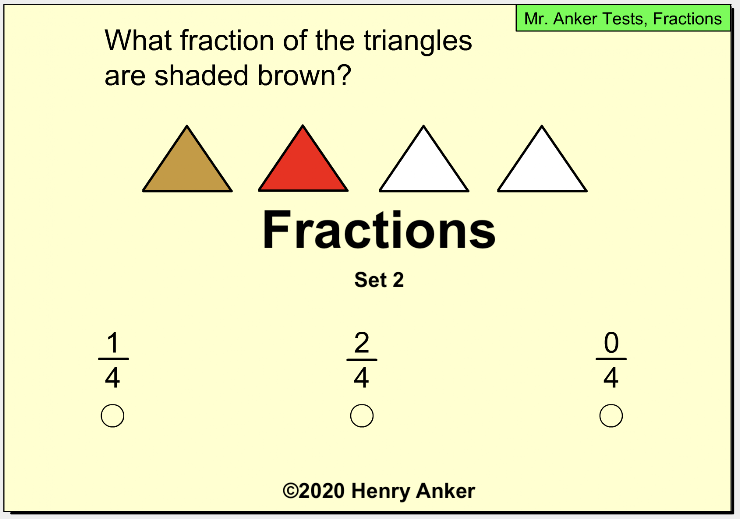1.  How much is shaded? *
1 point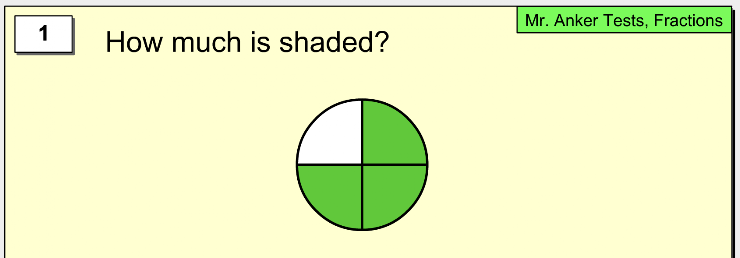2.  How much is shaded? *
1 point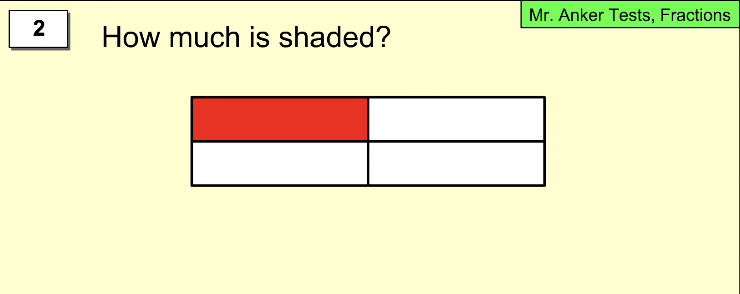3.  How much is shaded? *
1 point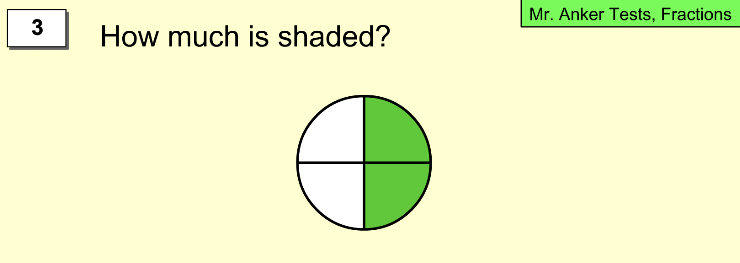4.  How much is shaded? *
1 point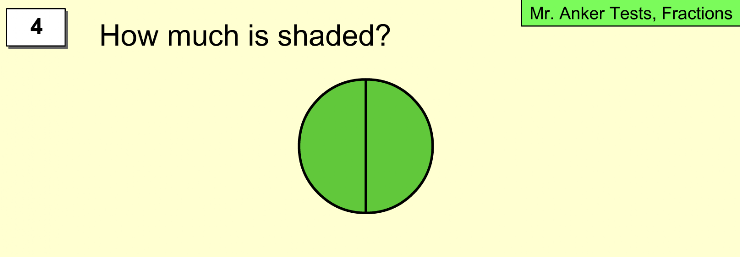5.  How much is shaded? *
1 point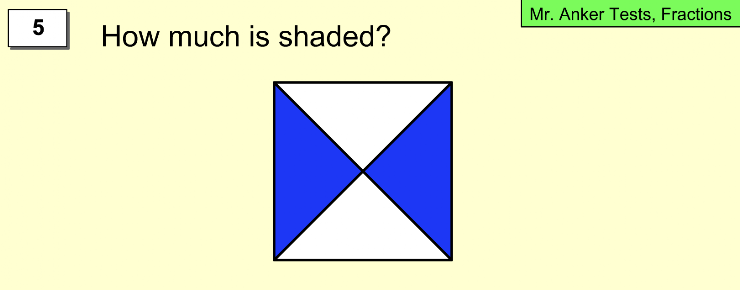6. How much is shaded? *
1 point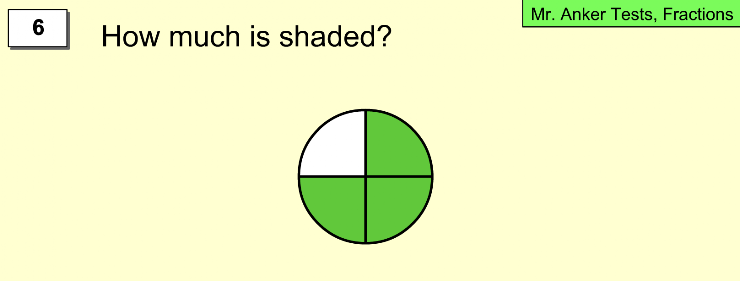7.  How much is shaded? *
1 point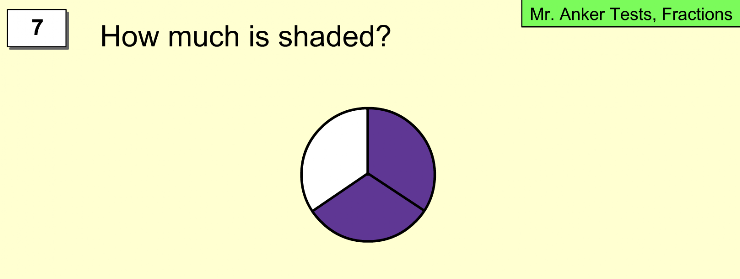8. How much is shaded? *
1 point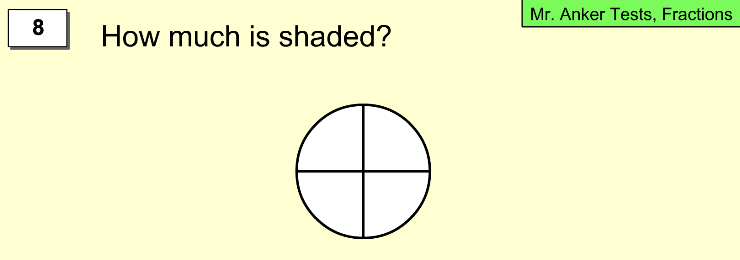9.  How much is shaded? *
1 point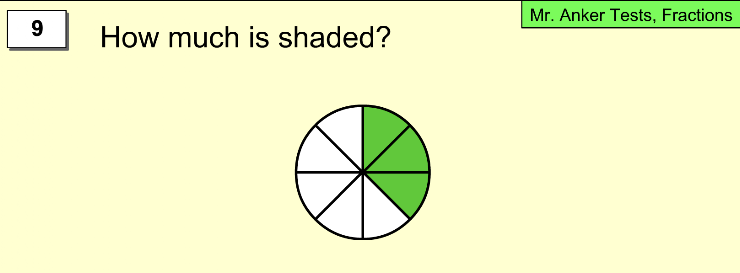10.  How much is shaded? *
1 point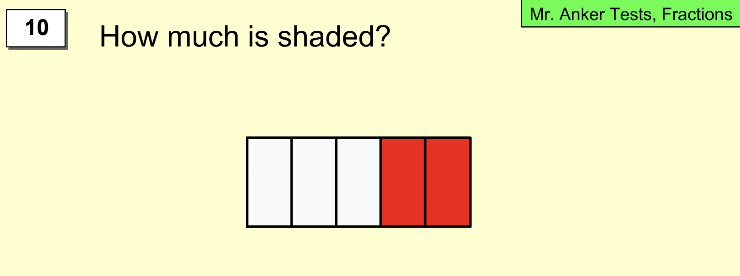11.  How much is shaded? *
1 point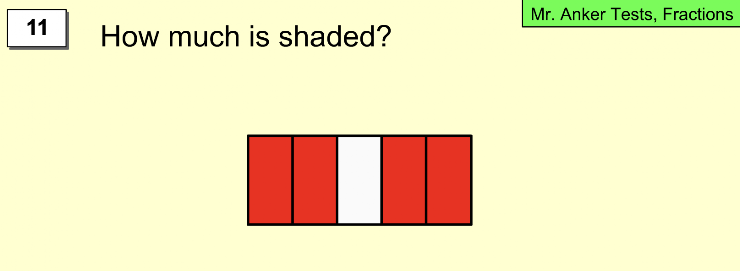12.  How much is shaded? *
1 point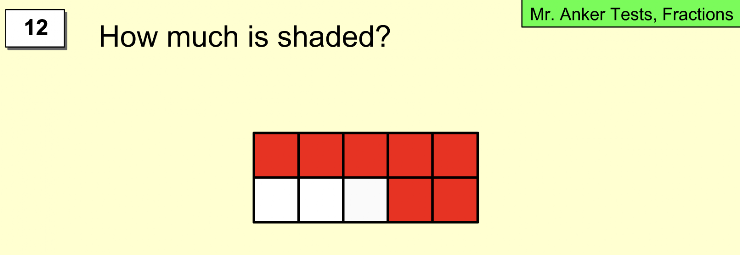13.  How much is shaded? *
1 point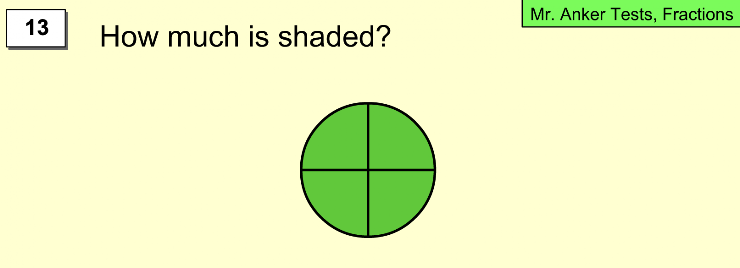14.  What fraction of the triangles are shaded brown? *
1 point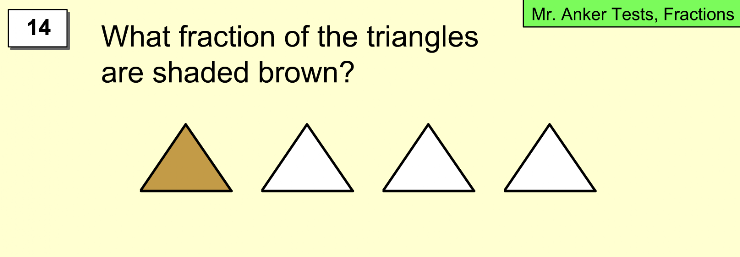15.  What fraction of the triangles are shaded brown? *
1 point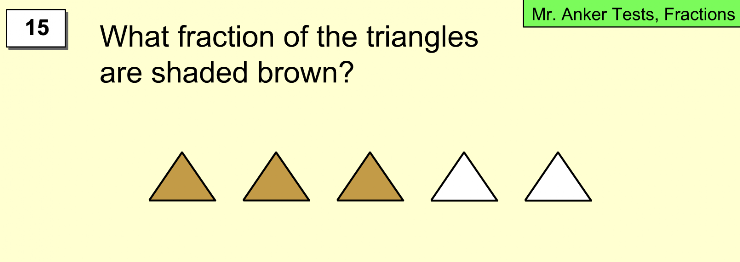16.  What fraction of the triangles are shaded brown? *
1 point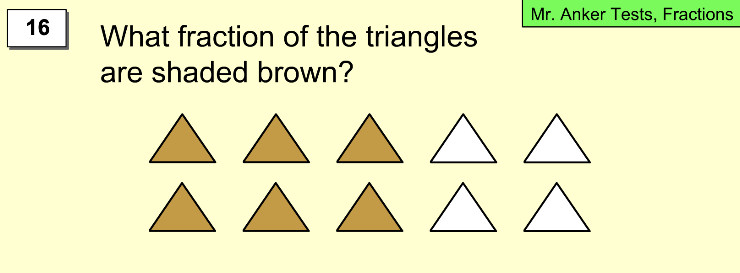17.  What fraction of the triangles are shaded brown? *
1 point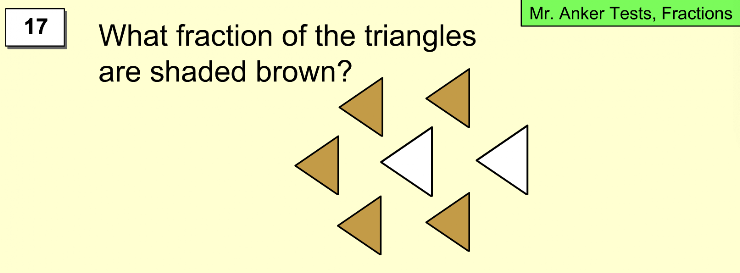18.  What fraction of the triangles are shaded brown? *
1 point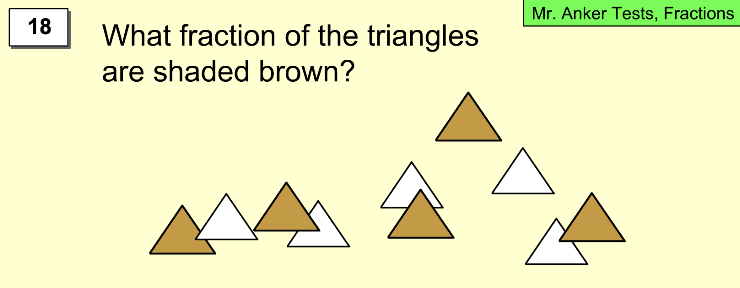19. What fraction of the cacti are shaded green? *
1 point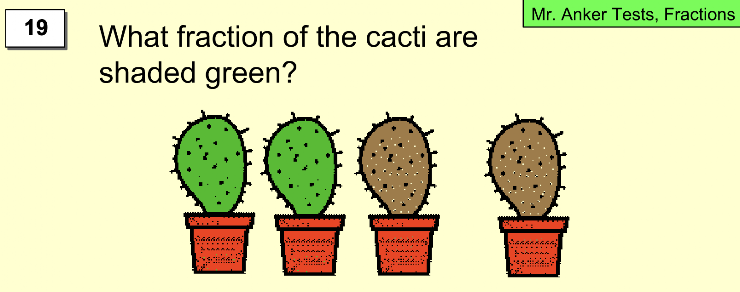20. What fraction of the pencils are yellow? *
1 point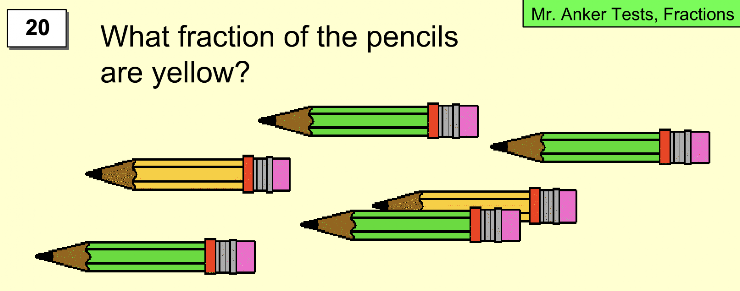Submit
Clear form